Editor Ratings:
User Ratings:
[Total: 5 Average: 4.2]

Mathcha is a free and powerful Math Editor, where you can write mathematical equations online. Not only mathematical equations, you can draw diagrams, draw graphs, inset tables, insert images, add table of contents, insert LaTex equations, and do much more. And all of this can be done very easily in this free math editor. You do not need to practice much or spend much time to know to use it. Just sign in to this online math editor using your Google or Facebook account and get going. You can create and save multiple documents with mathematical equations, and can save any equation as image, whenever required.

You can easily use LaTex equations here, or convert an equation to LaTex as well; however, writing mathematical equations on this online math editor is way easier than LaTex editors.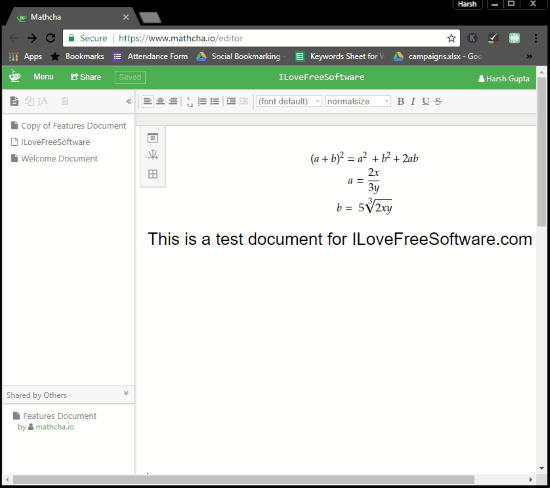## How To Write Mathematical Equations Online using Matcha?

As you open the Mathcha editor for the first time, you will be welcomed by a quick start guide. This guide tells you how to use this software to write equations, insert diagrams, insert tables, and more. Don’t worry; the guide is not too long, and finishes telling you what to do within 5 steps.

In order to write a mathematical equation, you need to first press \ button. This will bring up the menu to select what you want to write, draw, or insert. To write a mathematical equation along with text, you can select inline-math option, else you can select math-container to write equation in separate container. The same way, you can select any of the available options from the menu, which I will be explaining later.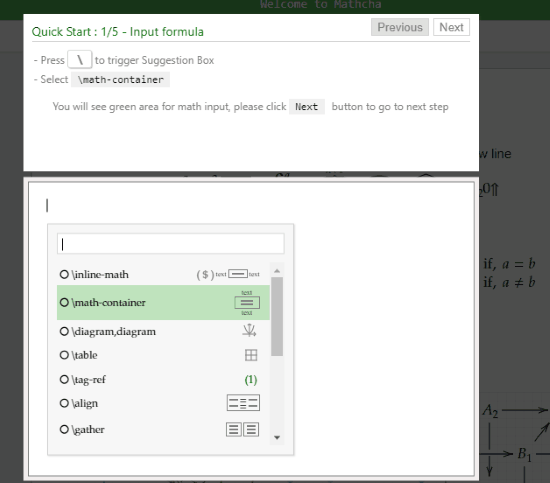After selecting inline-math or math-container, you can start writing math equations. To put power over a constant or variable, you can use ^ sign. To insert other mathematical symbols and expressions, simply press \ to trigger symbol suggestion box. Here, you can select from a long list of mathematical symbols, such as: square root, fraction, power, index, exclamation, percentage, underline, alpha, beta, hyphen, arrow, Euler, integration, and many more. You can scroll and search for these symbols, or start typing in the box to find symbol from its name.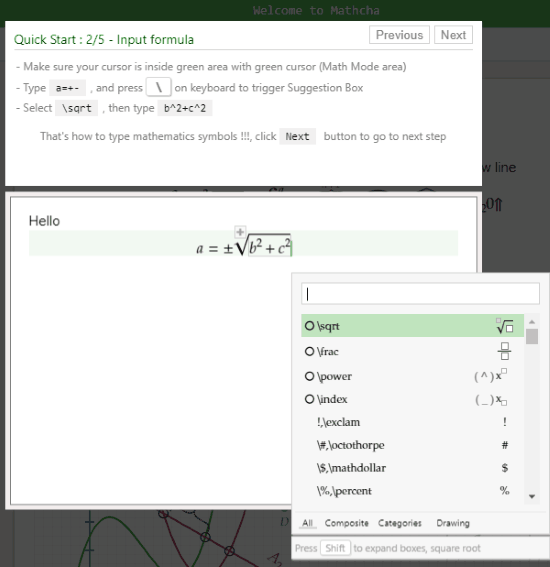The same way, you can add diagrams and graphs on the editor. For this, simply press \ and from the menu, select the diagram option. Then on the left side of the editor, you will observe a panel. From there, you can select the type of diagram you want to insert. You can insert, lines, circle, eclipse, square, rectangle, closed curve, axis, wave, arrow, etc. After adding an object, you can very easily modify its shape, size, position, or even color.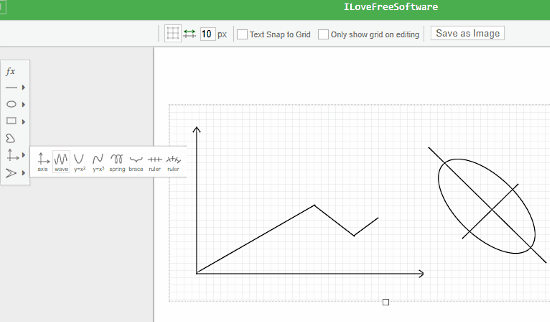To add tables, you will have to press \ button, then select the table option. This inserts a 3×3 table by default. Tables can be easily resized and you can also add or delete rows and columns in a table.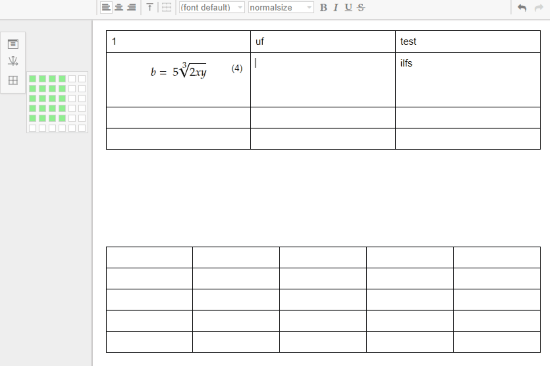As mentioned before, there are various other options to insert in the editor. Add Table of contents, image, tag reference, etc. Using these options are as easy as the options described above.

If you want, you can also save written mathematical formulas or diagrams as images. All you have to do is select the formula container or diagram, and click on the Save as Image option.

### Conclusion:

Mathcha is very powerful and easy to use online math editor. It can be really helpful for students or teachers to write mathematical equations online, then save or download them as images. You can even create multiple documents and access them whenever you want. Its support for LaTex is something I liked too much.